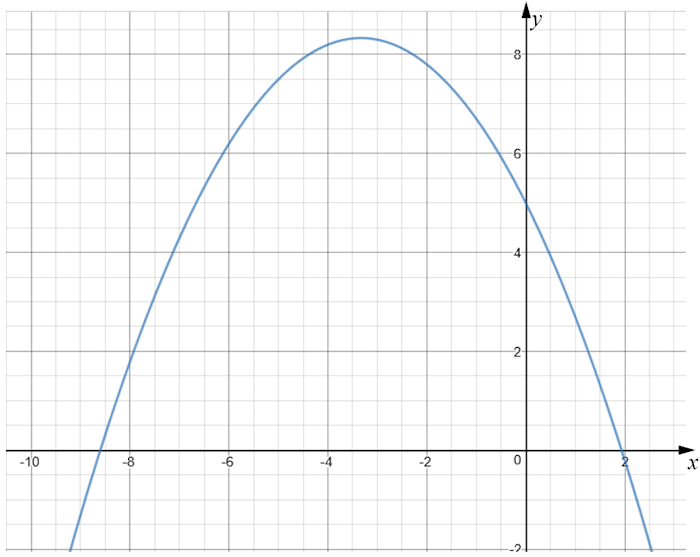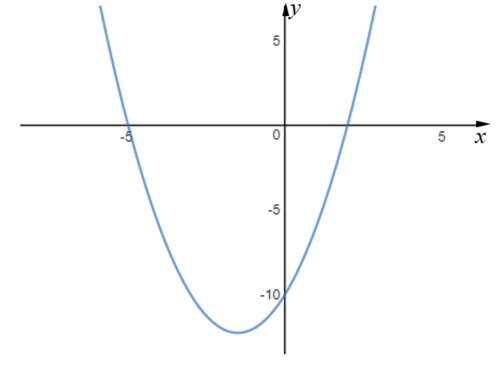# Exam-Style Questions.

## Problems adapted from questions set for previous Mathematics exams.

### 1.

GCSE Higher

The graph of y = f(x) is drawn accurately on the grid.

(a) Write down the coordinates of the turning point of the graph.(b) Write down estimates for the roots of f(x) = 0

(c) Use the graph to find an estimate for f(-5.5).

### 2.

GCSE Higher

(a) By completing the square, solve $$x^2+8x+13=0$$ giving your answer to three significant figures.

(b) From the completed square you found in part (a) find the minimum value of the curve $$y=x^2+8x+13$$.

### 3.

GCSE Higher

The diagram below is a sketch of a curve, a parabola, which is not drawn to scale.

The quadratic graph intersects the x-axis at (-5, 0) and at another point.

It also intersects the y-axis at (0, –10).

Work out the coordinates of the turning point of the graph if its equation is in the form $$y = x^2 + bx + c$$.### 4.

GCSE Higher

Given that $$x^2 – 8x + 3 = (x – a)^2 – b$$ for all values of x,

(a) find the value of a and the value of b.

(b) Hence write down the coordinates of the turning point on the graph of $$y = x^2 – 8x + 3$$

### 5.

GCSE Higher

(a) Find the interval for which $$x^2 - 9x + 18 \le 0$$

(b) The point (-4, -4) is the turning point of the graph of $$y = x^2 + ax + b$$, where a and b are integers. Find the values of a and b.

### 6.

GCSE Higher

(a) Write $$2x^2+8x+27$$ in the form $$a(x+b)^2+c$$ where $$a$$, $$b$$, and $$c$$ are integers, by 'completing the square'

(b) Hence, or otherwise, find the line of symmetry of the graph of $$y = 2x^2+8x+27$$

(c) Hence, or otherwise, find the turning point of the graph of $$y = 2x^2+8x+27$$

### 7.

IB Standard

A function is defined as $$f(x) = 2{(x - 3)^2} - 5$$ .

(a) Show that $$f(x) = 2{x^2} - 12x + 13$$.

(b) Write down the equation of the axis of symmetry of this graph.

(c) Find the coordinates of the vertex of the graph of $$f(x)$$.

(d) Write down the y-intercept.

(e) Make a sketch the graph of $$f(x)$$.

Let $$g(x) = {x^2}$$. The graph of $$f(x)$$ may be obtained from the graph of $$g(x)$$ by the two transformations:

• a stretch of scale factor $$s$$ in the y-direction;
• followed by a translation of $$\left( {\begin{array}{*{20}{c}} j\\ k \end{array}} \right)$$ .

(f) Find the values of $$j$$, $$k$$ and $$s$$.

### 8.

IB Standard

Let $$f(x)=5x^2-20x+k$$. The equation $$f(x)=0$$ has two equal roots.

(a) Write down the value of the discriminant.

(b) Hence, show that $$k=20$$.

The graph of $$f$$ has its vertex on the x-axis.

(c) Find the coordinates of the vertex of the graph of $$f$$.

(d) Write down the solution of $$f(x)=0$$.

The function can be written in the form $$f(x)=a(x-h)^2+j$$.

(e) Find the value of $$a$$.

(f) Find the value of $$h$$.

(g) Find the value of $$j$$.

(h) The graph of a function $$g$$ is obtained from the graph of $$f$$ by a reflection in the x-axis, followed by a translation by the vector $$\begin{pmatrix} 0 \\ 3 \\ \end{pmatrix}$$. Find $$g$$, giving your answer in the form $$g(x)=Ax^2+Bx+C$$.

If you would like space on the right of the question to write out the solution try this Thinning Feature. It will collapse the text into the left half of your screen but large diagrams will remain unchanged.

The exam-style questions appearing on this site are based on those set in previous examinations (or sample assessment papers for future examinations) by the major examination boards. The wording, diagrams and figures used in these questions have been changed from the originals so that students can have fresh, relevant problem solving practice even if they have previously worked through the related exam paper.

The solutions to the questions on this website are only available to those who have a Transum Subscription.

Exam-Style Questions Main Page

Search for exam-style questions containing a particular word or phrase:

To search the entire Transum website use the search box in the grey area below.